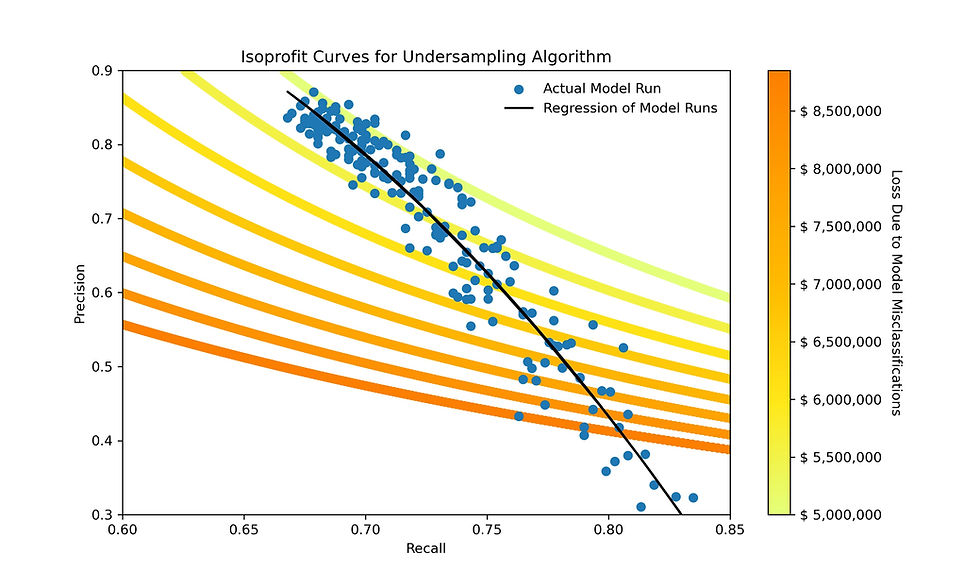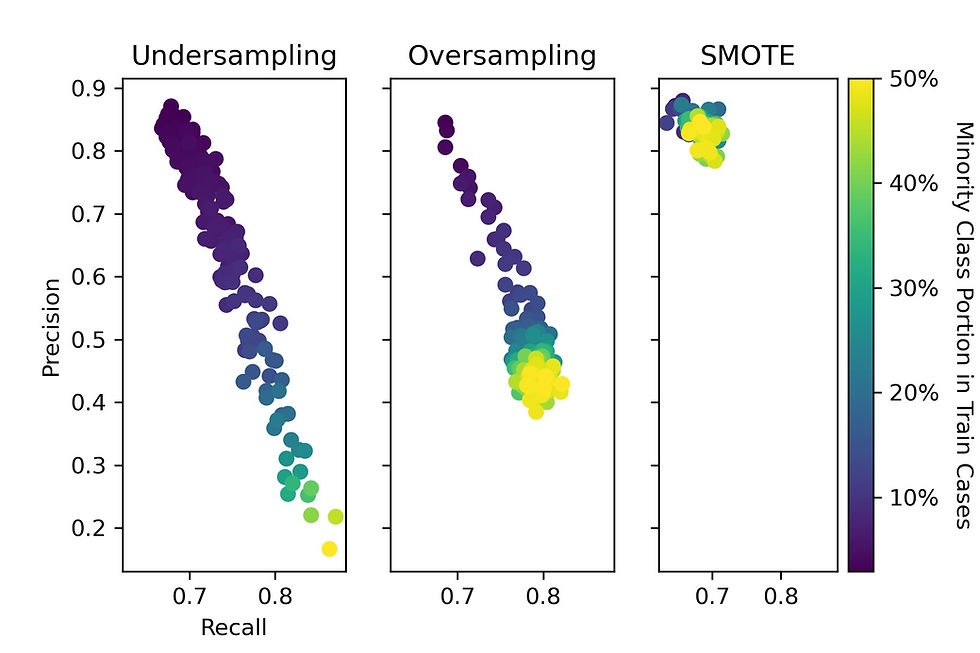top of page
Search
• Matt

# Managing Risk within Imbalanced Classes

Updated: Jul 2, 2020

"Rational people don’t risk what they have and need for what they don’t have and don’t need"

- Warren Buffett

Certain datasets suffer from class imbalance, the situation where one outcome is rare. Imbalanced data presents a challenge from a modeling perspective. Namely, the majority class outweighs the minority class and the model can over predict the majority outcome. In this analysis, we analyze three algorithms to overcome barriers on imbalanced data.

We are going to explore credit defaults for recently issued loans through the Lending Club platform. Around 2% of the loans are in default status, a significant class imbalance. If we assume all loans are good loans and try to predict credit default status, we immediately get an accuracy score of 98%. With imbalanced classes, it is often important to focus on predictions for the minority class (loans in default status).

Different types of misclassification errors have different costs. If we suspect that a loan will default and choose not to invest, we miss out on the potential return of interest rate payments over the life of the loan (\$8,000 on average per loan). However, if we predict that a loan will be good but it turns out to default later, we lose all our principle (\$17,000 on average per loan). Clearly if a loan had a 50% chance of defaulting, we would choose not to invest because the potential risk outweighs the potential return.

Based on my analysis, there is a tradeoff between precision and recall. We can balance the dataset by increasing the portion of minority cases or decreasing the portion of majority cases in the train data. These methods increase the recall (ability to predict positive cases) but decrease the precision (accuracy of positive case predictions).

This analysis leverages a multilayer perceptron (MLP) regression. The full code can be found on my GitHub.

### Data Sources

Lending Club is a peer to peer lending platform that publishes anonymized data on its loans. Fortunately for the lenders, most loans are repaid. On June 17th, 2020, I pulled data on more than 125,000 loans, totaling \$2.1B, written from October 1st to December 31st, 2019. Every loan in the data set has a 3 or 5 year term and has only been in existence from 169 to 260 days. Around 2% of those new loans are already past due. After an extract, transform, load process (ETL), we end up with over 100 features.

We converted the label ('loan_status') into a binary classification problem. A 'loan_status' of 'Charged Off', 'In Grace Period', 'Late (16-30 days)', or 'Late (31-120 days)' is considered to be in default status and was assigned a value of 1. A 'loan_status' of 'Current', 'Fully Paid', or 'Issued' is considered to be a good loan and was assigned a value of zero (98% of the cases).

### Analysis

We use a MLPClassifier neural network to predict if loans are in default status.

```# This model leverages the MLPClassifier neural network
mlp = MLPClassifier(hidden_layer_sizes=(10, 10), activation='logistic',
max_iter=2000)
mlp.fit(standardized, y_train)
predictions = mlp.predict(scaler.transform(x_test))```

The base case model has an accuracy of 99.0%. While this sounds impressive, it is important to remember that strictly predicting the majority class (all loans are good loans) yields an accuracy around 98%. To further explore prediction effectiveness for the minority class, we calculate precision and recall.

```precision = true_positives/(true_positives+false_positives)
recall = true_positives/(true_positives+false_negatives)```

Our base case precision is 84.5%; out of the 452 positive (default) predictions, 382 were actually positive. And our base case recall is 68.6%; out of the 557 positive (default) cases, 382 were correctly predicted. Within the test set, both types of misclassifications cost a combined \$3,215,929.

The first part of this analysis is going to seek to improve the model's recall. We used three algorithms to improve recall and overcome class imbalance.

1) Undersampling the majority class

2) Oversampling the minority class

3) The Synthetic Minority Over-sampling TEchnique (SMOTE)

Across all methods, it is critical to split the test and train data prior to analysis. We want to ensure the test data reflects the original dataset. We also set a random state to improve the consistency of our analysis.

`x_train, x_test, y_train, y_test = train_test_split(loans_base.drop('loan_status', axis=1),                                                                                                                               loans_base['loan_status'], test_size=0.2, random_state=42)`

Undersampling: Under this method, we remove majority class samples from the train set to balance the classes. Unfortunately, this method throws away perfectly good data that could improve our model. The graph below shows the impact of deleting majority class samples. As the bubble color shifts from purple to yellow, the dataset becomes more balanced. Our recall increases, but the precision drops significantly.

```# Undersampling method
drop_indices = np.random.choice(y_train[y_train==1].index,
remove_n, replace=False)
x_train = x_train.drop(drop_indices)
y_train = y_train.drop(drop_indices)
accuracy, recall, precision =
run_model(x_train, x_test, y_train, y_test)```Oversampling: Under this method, we resample (or 'double count') minority class samples from the train set to balance the classes. It is important to maintain separation from the test and train dataset. If we augment the training set with test set data, we risk overfitting the model. The graph below shows the impact of increasing the number of minority class samples. Our recall initially increases, but then plateaus.

```# Oversampling method
random_state=42).index
accuracy, recall, precision =
run_model(x_train, x_test, y_train, y_test)```SMOTE: Under this method, we add synthetically created minority class samples to the train set to balance the classes. New minority class samples are synthesized by creating points that are statistically similar to existing points. The graph below shows that the addition of new minority class samples slightly increased the model's recall without sacrificing precision.

```# SMOTE method
from imblearn.over_sampling import SMOTE
sm = SMOTE(sampling_strategy=ratio/100, random_state=42)
x_train, y_train = sm.fit_resample(x_train, y_train)
accuracy, recall, precision =
run_model(x_train, x_test, y_train, y_test)```Now that we have demonstrated ways to improve the model's recall (at the expensive of precision), we are going to evaluate the profit impact of each model. Because the undersampling algorithm showed a broader range of recall values, we focus subsequent analysis on that algorithm.

We are seeking the optimal balance between precision and recall. In order to model the profit impact of a precision-recall tradeoff, we need to make some assumptions. For this analysis, we assume that all negative loan terms (even those simply in the grace period) eventually default and result in the complete loss of principle. We also assume that lost opportunity cost (passing up a good loan opportunity) is the simple multiplication of principle, interest rate, loan term in years, and a 3% annual discount factor.

This analysis relies heavily on the base case (random_state = 42). We assume that the average default loss and the average lost opportunity costs are fixed. Each false_negative (loan we modeled to be good, but ends up defaulting) costs \$16,561. Each false_positive (good loan that we passed on) has an opportunity cost of \$7,748.

To quantify and evaluate the precision-recall tradeoff, we develop indifference curves. Indifference curves show points of equal 'profit'. Specifically goal is to minimize our objective function, losses due to false_positives and false_negatives within the test set. The blue dots in the chart below show model runs for the undersampling algorithm. The black line is a quadratic regression through those model runs. The yellow to orange colored lines are our isoprofit curves. Yellow indicates the lowest losses from the model. The \$5,000,000 isoprofit curve lies roughly tangential to the black line around recall = 0.7 and precision = 0.8. This means that "improvements" in recall do not result in more profitable models.### Conclusions and Potential Next StepsThe undersampling and oversampling algorithms show the tradeoff between precision and recall. Balancing the classes improves the likelihood that bad loans are identified, however there is a significant increase in the number good loans that are mistakenly identified as bad loans.

In this analysis, there was little to no financial benefit for balancing these classes.

1) Other class balancing algorithms including SMoteBoosting, borderline SMOTE, and ADASYN (Adaptive Synthetic Sampling Method for Imbalanced Data).

2) Evaluating the computational speed vs the precision/recall metrics for each algorithm. The undersampled model ends up training on 1,600 data points, while the oversampled model and the SMOTE model train on more than 165,000 points.

3) Incorporating the objective function directly into the neural network. MLPClassifier does not support custom log functions, but other neural networks would enable us to input the loan losses directly into the model.

4) Autoencoders are commonly used in fraud detection applications and imbalance classes.

166 views1 comment

See All
Post: Blog2_Post
bottom of page